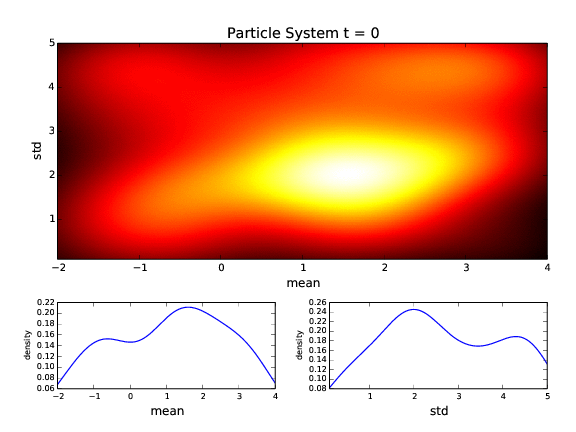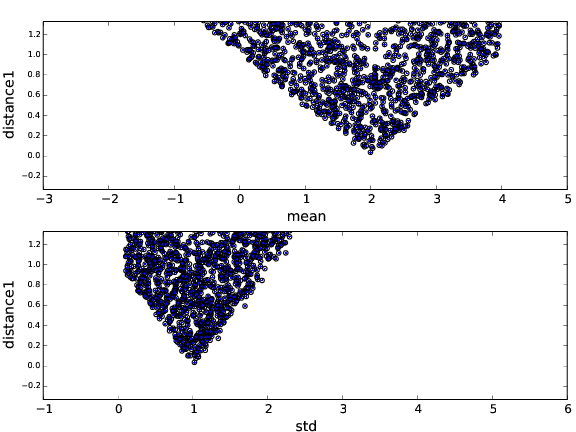# cosmoabc - Likelihood free inference for cosmology¶

`cosmoabc` is a package which enables parameter inference using an Approximate Bayesian Computation (ABC) algorithm, as described in Ishida et al., 2015.

The code was originally designed for cosmological parameter inference from galaxy clusters number counts based on Sunyaev-Zel’dovich measurements. In this context, the cosmological simulations were performed using the NumCosmo library .

Nevertheless, the user can easily take advantage of the ABC sampler along with his/her own simulator, as well as test personalized prior distributions and distance functions.

## Get it now!¶

The package can be installed using the PyPI and pip:

```\$ pip install cosmoabc
```

Or if the tarball or repository is downloaded, in the `cosmoabc` directory you can install and test it:

```\$ python setup.py install
```

You can run a few tests with:

```\$ test_ABC_algorithm.py
```

Warning

The above tests will generate a lot of output data file and a pdf file with their graphical representation. This was chosen to facilitate the identification of errors.

Make sure to run the tests in their own directory.

The test outputs a file illustrating the evolution of the posterior.## Input Parameter File¶

Sample input in can be found in `~cosmoabc/examples`. All example files mentioned in this section are available in that directory.

The user input file should contain all necessary variables for simulation as well as for the ABC sampler.

A simple example of user input file, using a simulator which takes 3 parameters as input (`mean`, `std`, `n`) from which we want to fit only two (`mean`, `std`), would look like this

```path_to_obs         = None                     # path to observed data

param_to_fit        = mean  std                # parameters to fit
param_to_sim        = mean  std  n             # parameters needed for simulation

prior_func          = my_prior flat_prior      # one prior function for each parameter
# under consideration

mean_prior_par_name      = pmin pmax           # parameters for prior distribution
mean_prior_par_val       = -2.0  4.0           # values for prior distribution

std_prior_par_name       = pmin  pmax          # parameters for prior distribution
std_prior_par_val        =  0.1  5.0           # values for prior distribution

mean_lim                 = -2.0  4.0           # extreme limits for parametes
std_lim                  = 0.1  5.0            #

mean                     = 2.0                 # parameters values need for simulation
std         = 1.0                              #
n           = 1000                             #

M           = 100                              # number of particle in each particle system
Mini        = 200                              # number of draws for 1st particle system
delta       = 0.25                             # convergence criteria
qthreshold  = 0.75                             # quantile in distance threshold

file_root       = toy_model_PS                 # root to output files names
screen          = 0                            # rather (1) or not (0) to screen outputs
ncores          = 1                            # number of cores
split_output    = 1                            # number of intermediate steps written to file

simulation_func = my_simulation                # simulation function
distance_func   = my_distance                  # distance function
```

### Important notes on input parameters¶

• In the current implementation, it is important to include a `` # `` symbol preceded and followed by a white space after the definition of each variable in the input file.
• The variable `path_to_obs` can be set to `None`, in this case, `cosmoabc` will generate one catalogue from the simulator and consider it as the observed catalogue. It will also output this catalogue to a data file, so it can be used for further scrutnity.
• If you are using your own prior function, you are free to name the prior parameters as you wish, you only need to define `<your_variable>_prior_par_name` and `<your_variable>_prior_par_val` accordingly. If you have doubts about variable names, a quick comparison between the two example input files (toy model and NumCosmo) might help.
• The parameter `split_output` determines how many sub-sets of particles you wish to generate for each particle system. Its goal is to avoid the lost of partial results for an enventual problem when dealing with very complex, and time consuming, simulators. If you are only making a quick test and has no intention to keep partial results, just set `split_output = 1`.

## Simulation, distance and prior functions¶

The most important ingredients in an ABC analysis are:

• the prior probability distributions (PDF)
• the simulator
• the distance function

Built-in options for priors PDF are:

• Gaussian
• flat
• beta

Built-in option for simulations is:

• NumCosmo simulation

Built-in options for distance functions are:

• Quantile-based distance with number of objects criteria
• Gaussian Radial Basis Function distance (as descrived in Appendix B of Ishida et al., 2015)

Moreover, `cosmoabc` is also able to handle user defined functions for all three elements. You will find example files which will help you tailor your functions for the ABC sampler.

Once all the function definitions are determined, the ABC sampler can be called from the command line:

```\$ run_ABC.py -i <user_input_file>  -f <user_function_file>
```

This will run the algorithm until the convergence criteria is reached. A pdf file containing graphical representation of the results for each particle system is given as output, as well as numerical data files.

If the achieved result is not satisfactory, or if for some reason the calculation was stopped before reaching the convergence criteria, it is possible to run the ABC sampler beginning from the last completed particle system `N`.

From the command line:

```\$ continue_ABC.py -i <user_input_file> -f <user_function_file> -p N
```

In case the convergence criteria was achieved but you wish to continue the run, remember to decrease the convergence criteria `delta` in the `<user_input_file>` before continuing.

At any time it is possible to plot the outcomes from `N` particle systems, whose calculations were completed, using:

```\$ plot_ABC.py -i <user_input_file> -p N
```

It is also possible to use it interactively. Considering we are using built-in simulation, prior and distance functions,

```from cosmoabc.priors import flat_prior
from cosmoabc.ABC_sampler import ABC
from cosmoabc.plots import plot_2p
import numpy as np

#user input file
filename = 'user.input'

#initiate ABC sampler
sampler_ABC = ABC(params=Parameters)

#build first particle system
sys1 = sampler_ABC.BuildFirstPSystem()

#update particle system until convergence
sampler_ABC.fullABC()

#plot results
plot_2p( sampler_ABC.T, 'results.pdf' , Parameters)
```

If you are using your own distance function, remember to update the dictionary of parameters and determine the dimension of its output manually,

```from cosmoabc.priors import flat_prior
from cosmoabc.ABC_sampler import ABC
from cosmoabc.plots import plot_2p
import numpy as np

from my_functions import my_distance, my_sim, my_prior

#user input file
filename = 'user.input'

# update dictionary of user input parameters
Parameters['distance_func'] = my_distance
Parameters['simulation_func'] = my_sim

# update the dictionary of prior parameters for each parameter
Parameters['prior']['mean']['func'] = my_prior

# in case you want to generate a pseudo-observed data set
Parameters['dataset1'] = my_sim(Parameters['simulation_input'])

#calculate distance between 2 catalogues
dtemp = my_distance(Parameters['dataset1'], Parameters)

#determine dimension of distance output
Parameters['dist_dim'] = len(dtemp)

#initiate ABC sampler
sampler_ABC = ABC(params=Parameters)

#build first particle system
sys1 = sampler_ABC.BuildFirstPSystem()

#update particle system until convergence
sampler_ABC.fullABC()

#plot results
plot_2p( sampler_ABC.T, 'results.pdf' , params)
```

Warning

When using your own distance function remember that it must take as input:
- a catalogue and
- a dictionary of input parameters

When using your own prior function, it must take as input:
- a dictionary of input parameters
- a boolean variable `func` (optional):
if `func` is `False` returns one sampling of the underlying distribution
if `func` is `True` returns the PDF itself

### NumCosmo simulations¶

In order to reproduce the results of Ishida et al., 2015, first you need to make sure the NumCosmo library is running smoothly. Instructions for complete installation and tests can be found at the NumCosmo website.

An example of input file for NumCosmo simulations is provided in the corresponding directory. Once the simulator is installed run the complete ABC sampler + NumCosmo cluster simulations from the command line:

```\$ run_ABC_NumCosmo.py -i <user_input_file>
```

This will run the complete analysis presented in the above paper.

Analogously to what is available for the user defined simulations, we can also continue a NumCosmo calculation from particle system `N` with:

```\$ continue_ABC_NumCosmo.py -i <user_input_file> -p N
```

If we want to run the NumCosmo simulation with a different prior or distance function, we should define it in a separate file and run:

```\$ run_ABC_NumCosmo.py -i <user_input_file> -f <user_function_file>
```

Plots are generated exactly as explained above for the user defined functions.

## Testing Distances¶

If you are using a personalized distance, make sure that it applies to the particular problem you are facing. You need to be sure that the distance definition you adpoted yields increasingly larger distances for increasingly different catalogues.

`cosmoabc` has a built-in script which allows you to visually test the performances of your choices. In order to use it, prepare an appropriate user input and function files and, from the command line, do:

```\$ test_ABC_distance.py -i <user_input_file> -f <user_function_file> -o <output_filename>
```

Here, `<output_filename>` is where the distance behaviour for different set of parameter values will be plotted.

As always, the `<user_input_file>` must be provided. If you are using built-in `cosmoabc` functions, the `-f` option is not necessary and in case you forget to give an output filename, `cosmoabc` will ask you for it. It will also ask you to input the number of points to be drawn from the parameter space in order to construct a grid.

Here is an example from using the built-in tool to check the suitability of the distance function described in section 3.1 of the paper:

```\$ test_ABC_distance.py -i user_input_file.dat -f user_function_file.dat
\$ Distance between identical cataloges = [ 0.]
\$ New parameter value = [ 0.41054026  0.6364732 ]
\$ Distance between observed and simulated data = [804.38711094885957]
\$ Enter number of draws in parameter grid: 5000
\$ Particle index: 1
\$ Particle index: 2
\$ Particle index: 3
\$ Particle index: 4
\$ ...
\$ Particle index: 5000
\$ Figure containing distance results is stored in output.pdf
```

The output file will contain a plot like this:The example above corresponds to a perfect distance definition, since it gets close to zero as parameters `mean` and `std` approaches the fiducial values and sharply increases for further values.

This is what one should aim for in constructing a distance function. How large a deviation from this is acceptable should be decided based on each particular problem and goal.

## Bibtex entry¶

If you use `cosmoabc` in you research, we kindly ask you to cite the original paper. The code includes a built-in citation function which outputs the bibtex entry

```import cosmoabc

cosmoabc.__cite__()
```

this will return:

```@ARTICLE{2015A&C....13....1I,
author = {{Ishida}, E.~E.~O. and {Vitenti}, S.~D.~P. and {Penna-Lima}, M. and
{Cisewski}, J. and {de Souza}, R.~S. and {Trindade}, A.~M.~M. and
{Cameron}, E. and {Busti}, V.~C.},
title = "{COSMOABC: Likelihood-free inference via Population Monte Carlo Approximate Bayesian Computation}",
journal = {Astronomy and Computing},
archivePrefix = "arXiv",
eprint = {1504.06129},
keywords = {Galaxies: statistics, (cosmology:) large-scale structure of universe},
year = 2015,
month = nov,
volume = 13,
pages = {1-11},
doi = {10.1016/j.ascom.2015.09.001},
adsnote = {Provided by the SAO/NASA Astrophysics Data System}
```

## Requirements¶

• Python 2.7
• numpy >=1.8.2
• scipy >= 0.14.0
• statsmodels >= 0.5.0
• matplotlib >= 1.3.1
• argparse >= 1.1
• multiprocessing >= 0.70a1

### Optional¶

• GNU General Public License (GPL>=3)

## The Cosmostatistics Initiative (COIN)¶

The IAA Cosmostatistics Initiative (COIN) is a non-profit organization whose aim is to nourish the synergy between astrophysics, cosmology, statistics and machine learning communities. This work is a product of the first COIN Residence Program, Lisbon, August/2014.

Other projects developed under COIN can be found in the COIN Portifolio.

## Acknowledgements¶

In order to give proper credit to the online sources used in the development of this work, we list bellow the main web websites, foruns and blogs which were used in different parts of its development. We deeply thank everyone who contributes to open learning plataforms.

Multiprocessing and KeyboarInterrupt

Ordering gif

Plotting

Remembering git commands